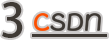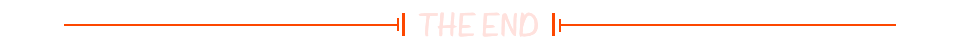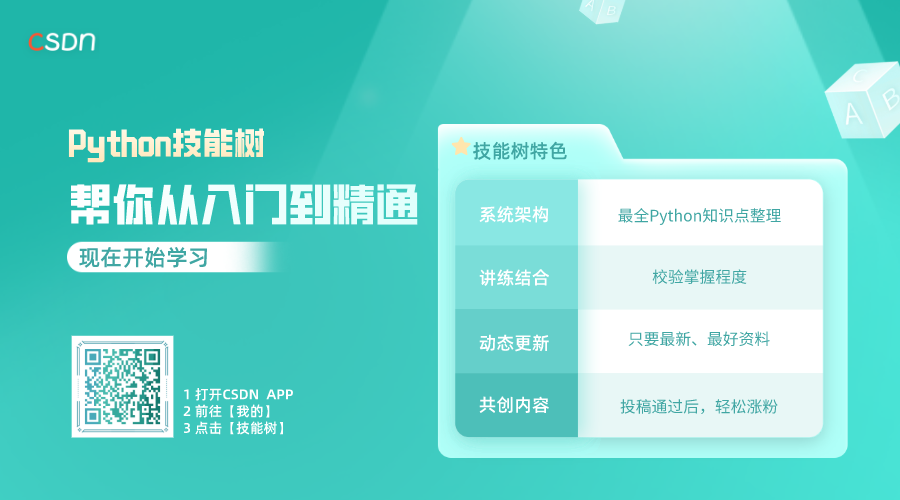#### 越来越难？这届开发者学不会的计算机理论

CSDN 2021-11-26 15:22Quake3的0x5f3759df 来自于应用界的最后尊严

https://github.com/raspberrypi/quake3/blob/8d89a2a3c1707bf0f75b2ea26645b872e97c0b95/code/qcommon/q_math.c

``float Q_rsqrt( float number )``{``        floatint_tt;``        floatx2, y;``        constfloat threehalfs = 1.5F;``        x2= number * 0.5F;``        t.f  = number;``        t.i  = 0x5f3759df - ( t.i >> 1 );               // what the fuck?``        y  = t.f;``        y  = y * ( threehalfs - ( x2 * y * y ) );   // 1st iteration``//     y  = y * ( threehalfs - ( x2 * y * y ) );   // 2nd iteration, this can be removed`` ``        returny;``}``

Quake3使用的0x5f3759df属于一个魔法数字。它来自平方根倒数速算法，在《Quake 3》建模引擎中引用这个魔法之后，其速度要比标准的牛顿迭代法快上4倍。

``i = 0x5f3759df - ( i >> 1 ); // what the fuck?```☞腾讯回应“腾讯云数据库泄露”传闻；特斯拉将推出13万元刹车套件；PHP 8.1.0正式发布 | 极客头条☞做开源数据库，九章云极DataCanvas是认真的！☞技术“摸鱼” 5 年，国外小哥白拿 45 万工资！`